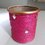# Complex Networks 4

(It is assumed that the reader has read the third article of this series)

As promised in the last article, in this article we will derive the exact form of $P(k)$, the degree distribution, for ER network. To derive the exact form of $P(k)$, observe that if a certain node is connected to given $k$ other nodes, then probability of this event is $p^{k}(1-p)^{N-1-k}$ where $p$ the linking probability as defined in the first article and $N$ is the total number of nodes in the network. Let me explain this in some detail. Since the probability that our chosen node is connected to one other node is $p$, the probability that it is not connected to that node is $(1-p)$. Out of total $N-1$ nodes which this node can connect to, it is connected to $k$ nodes so this gives us factor $p^{k}$. This also means that our node is not connected to $N-1-k$ nodes which gives us factor of $(1-p)^{N-1-k}$. But this calculation we did for 'given' $k$ number of nodes. But observe that we can actually choose these $k$ nodes from remaining $N-1$ nodes in $^{N-1}C_{k}$ ways. This gives us:

$P(k)=^{N-1}C_{k}p^{k}(1-p)^{N-1-k}$

Just to simplify this expression, let us assume that we there were $N+1$ number of nodes to start with instead of $N$. Then we get:

$P(k)=^{N}C_{k}p^{k}(1-p)^{N-k}$

Does this expression resemble something? Consider expansion of $(x+y)^{N}$ and you will realize that $P(k)$ is nothing but a binomial distribution!. Now we want to see what happens if we increase the size of the network. As we increase the number of nodes, in general, we don't expect that average degree of the nodes would increase. For example, if the number of people in the city increases by $2$, we don't expect that average number of friends of person living in that city would double. Thus we arrive at an important conclusion that instead of $p$, we should really keep $z$, the average degree of the network, a parameter to describe ER graph. Hence to study larger and larger ER networks, we first keep average degree of the network constant and then see what happens as the network grows. As was mentioned in previous articles, for ER network, $z=pN$ and hence while simulating larger and larger ER networks, we must decrease $p$ appropriately so as to keep $z$ constant.

Now let us rewrite $P(k)$ using $z$ as the parameter instead of $p$. After some algebraic manipulations,in the limit of large $N$, we get,

$P(k)=\frac{z^{k}e^{-z}}{K!}$

This is called a Poisson distribution. You can see the whole derivation here. Thus we see that ER network has Poisson distribution for degrees and this fact is going to be very important for our future journey.

In the next article we will try to look deep into the structure of social networks and we will discover an extremely important property of them which will ultimately be related to ER network. So be ready! :-)Note by Snehal Shekatkar
7 years ago

This discussion board is a place to discuss our Daily Challenges and the math and science related to those challenges. Explanations are more than just a solution — they should explain the steps and thinking strategies that you used to obtain the solution. Comments should further the discussion of math and science.

When posting on Brilliant:

• Use the emojis to react to an explanation, whether you're congratulating a job well done , or just really confused .
• Ask specific questions about the challenge or the steps in somebody's explanation. Well-posed questions can add a lot to the discussion, but posting "I don't understand!" doesn't help anyone.
• Try to contribute something new to the discussion, whether it is an extension, generalization or other idea related to the challenge.

MarkdownAppears as
*italics* or _italics_ italics
**bold** or __bold__ bold
- bulleted- list
• bulleted
• list
1. numbered2. list
1. numbered
2. list
Note: you must add a full line of space before and after lists for them to show up correctly
paragraph 1paragraph 2

paragraph 1

paragraph 2

[example link](https://brilliant.org)example link
> This is a quote
This is a quote
    # I indented these lines
# 4 spaces, and now they show
# up as a code block.

print "hello world"
# I indented these lines
# 4 spaces, and now they show
# up as a code block.

print "hello world"
MathAppears as
Remember to wrap math in $$ ... $$ or $ ... $ to ensure proper formatting.
2 \times 3 $2 \times 3$
2^{34} $2^{34}$
a_{i-1} $a_{i-1}$
\frac{2}{3} $\frac{2}{3}$
\sqrt{2} $\sqrt{2}$
\sum_{i=1}^3 $\sum_{i=1}^3$
\sin \theta $\sin \theta$
\boxed{123} $\boxed{123}$

Sort by:

Thank you for sharing your research! Somehow I feel that networks have enormous power and capabilities to enrich our understanding of the variety of compex phenomena around us; and all major discoveries in this field are to be made in the future.

Good news! The course "Social and economic networks" has started on coursera.org just a couple of hours ago, so all those willing to participate can sign up at any moment. (It's absolutely free.) I'm gonna try my best to learn everything I need concerning network and its applications,especially in social sciences. I have a special desire to learn more about social networks, therefore, I'm waiting for "Complex Networks 5 by Snehal Shekatkar" also.

- 7 years ago

Friends, please find the next article here.

- 7 years ago